{% raw %}

# 十六、使用 RNN 和注意力机制进行自然语言处理

## 使用 Character RNN 生成莎士比亚风格的文本

PANDARUS:
Alas, I think he shall be come approached and the day
When little srain would be attain'd into being never fed,
And who is but a chain and subjects of his death,
I should not sleep.


### 创建训练数据集

shakespeare_url = "https://homl.info/shakespeare" # shortcut URL
filepath = keras.utils.get_file("shakespeare.txt", shakespeare_url)
with open(filepath) as f:


tokenizer = keras.preprocessing.text.Tokenizer(char_level=True)
tokenizer.fit_on_texts([shakespeare_text])


>>> tokenizer.texts_to_sequences(["First"])
[[20, 6, 9, 8, 3]]
>>> tokenizer.sequences_to_texts([[20, 6, 9, 8, 3]])
['f i r s t']
>>> max_id = len(tokenizer.word_index) # number of distinct characters
>>> dataset_size = tokenizer.document_count # total number of characters


[encoded] = np.array(tokenizer.texts_to_sequences([shakespeare_text])) - 1


### 如何切分序列数据集

train_size = dataset_size * 90 // 100
dataset = tf.data.Dataset.from_tensor_slices(encoded[:train_size])


### 将序列数据集切分成多个窗口

n_steps = 100
window_length = n_steps + 1 # target = input 向前移动 1 个字符
dataset = dataset.window(window_length, shift=1, drop_remainder=True)


window()方法创建了一个包含窗口的数据集，每个窗口也是数据集。这是一个嵌套的数据集，类似于列表的列表。当调用数据集方法处理（比如、打散或做批次）每个窗口时，这样会很方便。但是，不能直接使用嵌套数据集来训练，因为模型要的输入是张量，不是数据集。因此，必须调用flat_map()方法：它能将嵌套数据集转换成打平的数据集。例如，假设{1, 2, 3}表示包含张量 1、2、3 的序列。如果将嵌套数据集{{1, 2}, {3, 4, 5, 6}}打平，就会得到{1, 2, 3, 4, 5, 6}。另外，flat_map()方法可以接收函数作为参数，可以处理嵌套数据集的每个数据集。例如，如果将函数 lambda ds: ds.batch(2) 传递给 flat_map() ，它能将{{1, 2}, {3, 4, 5, 6}}转变为{[1, 2], [3, 4], [5, 6]}：这是一个张量大小为 2 的数据集。

dataset = dataset.flat_map(lambda window: window.batch(window_length))


batch_size = 32
dataset = dataset.shuffle(10000).batch(batch_size)
dataset = dataset.map(lambda windows: (windows[:, :-1], windows[:, 1:]))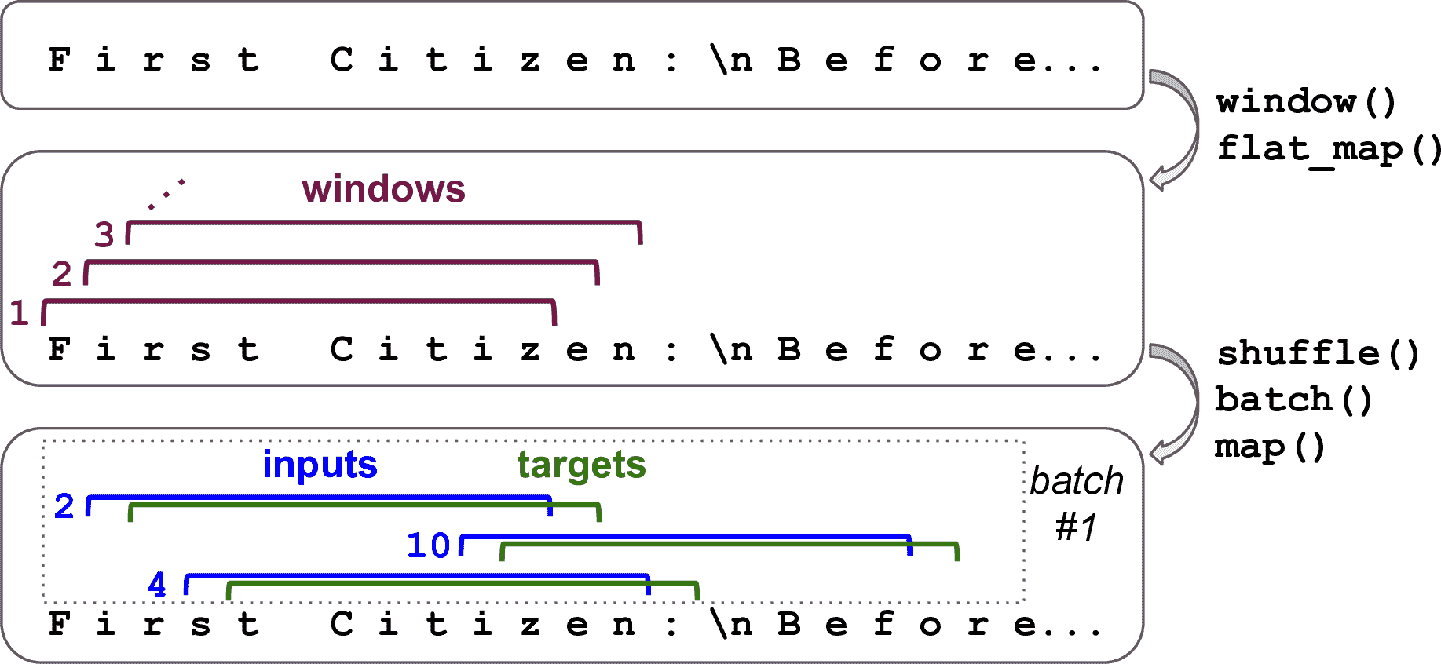dataset = dataset.map(
lambda X_batch, Y_batch: (tf.one_hot(X_batch, depth=max_id), Y_batch))


dataset = dataset.prefetch(1)


### 搭建并训练 Char-RNN 模型

model = keras.models.Sequential([
keras.layers.GRU(128, return_sequences=True, input_shape=[None, max_id],
dropout=0.2, recurrent_dropout=0.2),
keras.layers.GRU(128, return_sequences=True,
dropout=0.2, recurrent_dropout=0.2),
keras.layers.TimeDistributed(keras.layers.Dense(max_id,
activation="softmax"))
])
history = model.fit(dataset, epochs=20)


### 使用 Char-RNN 模型

def preprocess(texts):
X = np.array(tokenizer.texts_to_sequences(texts)) - 1
return tf.one_hot(X, max_id)


>>> X_new = preprocess(["How are yo"])
>>> Y_pred = model.predict_classes(X_new)
>>> tokenizer.sequences_to_texts(Y_pred + 1)[-1] # 1st sentence, last char
'u'


### 生成假莎士比亚文本

def next_char(text, temperature=1):
X_new = preprocess([text])
y_proba = model.predict(X_new)[0, -1:, :]
rescaled_logits = tf.math.log(y_proba) / temperature
char_id = tf.random.categorical(rescaled_logits, num_samples=1) + 1


def complete_text(text, n_chars=50, temperature=1):
for _ in range(n_chars):
text += next_char(text, temperature)
return text


>>> print(complete_text("t", temperature=0.2))
the belly the great and who shall be the belly the
>>> print(complete_text("w", temperature=1))
thing? or why you gremio.
who make which the first
>>> print(complete_text("w", temperature=2))
th no cce:
fol rusb


### 有状态 RNN

dataset = tf.data.Dataset.from_tensor_slices(encoded[:train_size])
dataset = dataset.window(window_length, shift=n_steps, drop_remainder=True)
dataset = dataset.flat_map(lambda window: window.batch(window_length))
dataset = dataset.batch(1)
dataset = dataset.map(lambda windows: (windows[:, :-1], windows[:, 1:]))
dataset = dataset.map(
lambda X_batch, Y_batch: (tf.one_hot(X_batch, depth=max_id), Y_batch))
dataset = dataset.prefetch(1)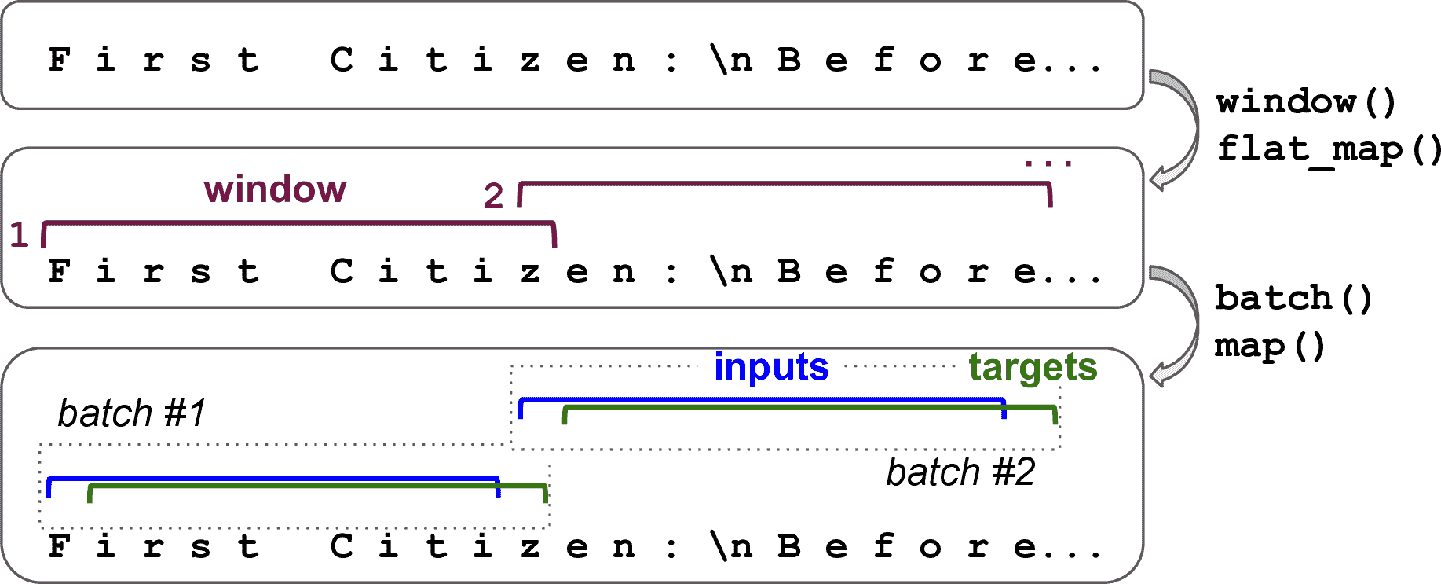model = keras.models.Sequential([
keras.layers.GRU(128, return_sequences=True, stateful=True,
dropout=0.2, recurrent_dropout=0.2,
batch_input_shape=[batch_size, None, max_id]),
keras.layers.GRU(128, return_sequences=True, stateful=True,
dropout=0.2, recurrent_dropout=0.2),
keras.layers.TimeDistributed(keras.layers.Dense(max_id,
activation="softmax"))
])


class ResetStatesCallback(keras.callbacks.Callback):
def on_epoch_begin(self, epoch, logs):
self.model.reset_states()


model.compile(loss="sparse_categorical_crossentropy", optimizer="Adam")
model.fit(dataset, epochs=50, callbacks=[ResetStatesCallback()])


## 情感分析

>>> (X_train, y_train), (X_test, y_test) = keras.datasets.imdb.load_data()
>>> X_train[:10]
[1, 14, 22, 16, 43, 530, 973, 1622, 1385, 65]


>>> word_index = keras.datasets.imdb.get_word_index()
>>> id_to_word = {id_ + 3: word for word, id_ in word_index.items()}
>>> for id_, token in enumerate(("<pad>", "<sos>", "<unk>")):
...     id_to_word[id_] = token
...
>>> " ".join([id_to_word[id_] for id_ in X_train[:10]])
'<sos> this film was just brilliant casting location scenery story'


import tensorflow_datasets as tfds

datasets, info = tfds.load("imdb_reviews", as_supervised=True, with_info=True)
train_size = info.splits["train"].num_examples


def preprocess(X_batch, y_batch):
X_batch = tf.strings.substr(X_batch, 0, 300)
X_batch = tf.strings.regex_replace(X_batch, b"<br\\s*/?>", b" ")
X_batch = tf.strings.regex_replace(X_batch, b"[^a-zA-Z']", b" ")
X_batch = tf.strings.split(X_batch)


from collections import Counter
vocabulary = Counter()
for X_batch, y_batch in datasets["train"].batch(32).map(preprocess):
for review in X_batch:
vocabulary.update(list(review.numpy()))


>>> vocabulary.most_common()[:3]
[(b'<pad>', 215797), (b'the', 61137), (b'a', 38564)]


vocab_size = 10000
truncated_vocabulary = [
word for word, count in vocabulary.most_common()[:vocab_size]]


words = tf.constant(truncated_vocabulary)
word_ids = tf.range(len(truncated_vocabulary), dtype=tf.int64)
vocab_init = tf.lookup.KeyValueTensorInitializer(words, word_ids)
num_oov_buckets = 1000
table = tf.lookup.StaticVocabularyTable(vocab_init, num_oov_buckets)


>>> table.lookup(tf.constant([b"This movie was faaaaaantastic".split()]))
<tf.Tensor: [...], dtype=int64, numpy=array([[   22,    12,    11, 10054]])>


def encode_words(X_batch, y_batch):
return table.lookup(X_batch), y_batch

train_set = datasets["train"].batch(32).map(preprocess)
train_set = train_set.map(encode_words).prefetch(1)


embed_size = 128
model = keras.models.Sequential([
keras.layers.Embedding(vocab_size + num_oov_buckets, embed_size,
input_shape=[None]),
keras.layers.GRU(128, return_sequences=True),
keras.layers.GRU(128),
keras.layers.Dense(1, activation="sigmoid")
])

metrics=["accuracy"])
history = model.fit(train_set, epochs=5)


### 遮掩

K = keras.backend
inputs = keras.layers.Input(shape=[None])
mask = keras.layers.Lambda(lambda inputs: K.not_equal(inputs, 0))(inputs)
z = keras.layers.Embedding(vocab_size + num_oov_buckets, embed_size)(inputs)
outputs = keras.layers.Dense(1, activation="sigmoid")(z)
model = keras.Model(inputs=[inputs], outputs=[outputs])


### 复用预训练的词嵌入

import tensorflow_hub as hub

model = keras.Sequential([
dtype=tf.string, input_shape=[], output_shape=),
keras.layers.Dense(128, activation="relu"),
keras.layers.Dense(1, activation="sigmoid")
])
metrics=["accuracy"])


hub.KerasLayer从给定的 URL 下载模块。这个特殊的模块是“句子编码器”：它接收字符串作为输入，将每句话编码为一个独立向量（这个例子中是 50 维度的向量）。在内部，它将字符串解析（空格分隔），然后使用预训练（训练语料是 Google News 7B，一共有 70 亿个词）的嵌入矩阵来嵌入每个词。然后计算所有词嵌入的平均值，结果是句子嵌入。我们接着可以添加两个简单的紧密层来创建一个出色的情感分析模型。默认，hub.KerasLayer是不可训练的，但创建时可以设定trainable=True，就可以针对自己的任务微调了。

datasets, info = tfds.load("imdb_reviews", as_supervised=True, with_info=True)
train_size = info.splits["train"].num_examples
batch_size = 32
train_set = datasets["train"].batch(batch_size).prefetch(1)
history = model.fit(train_set, epochs=5)


## 用编码器-解码器做机器翻译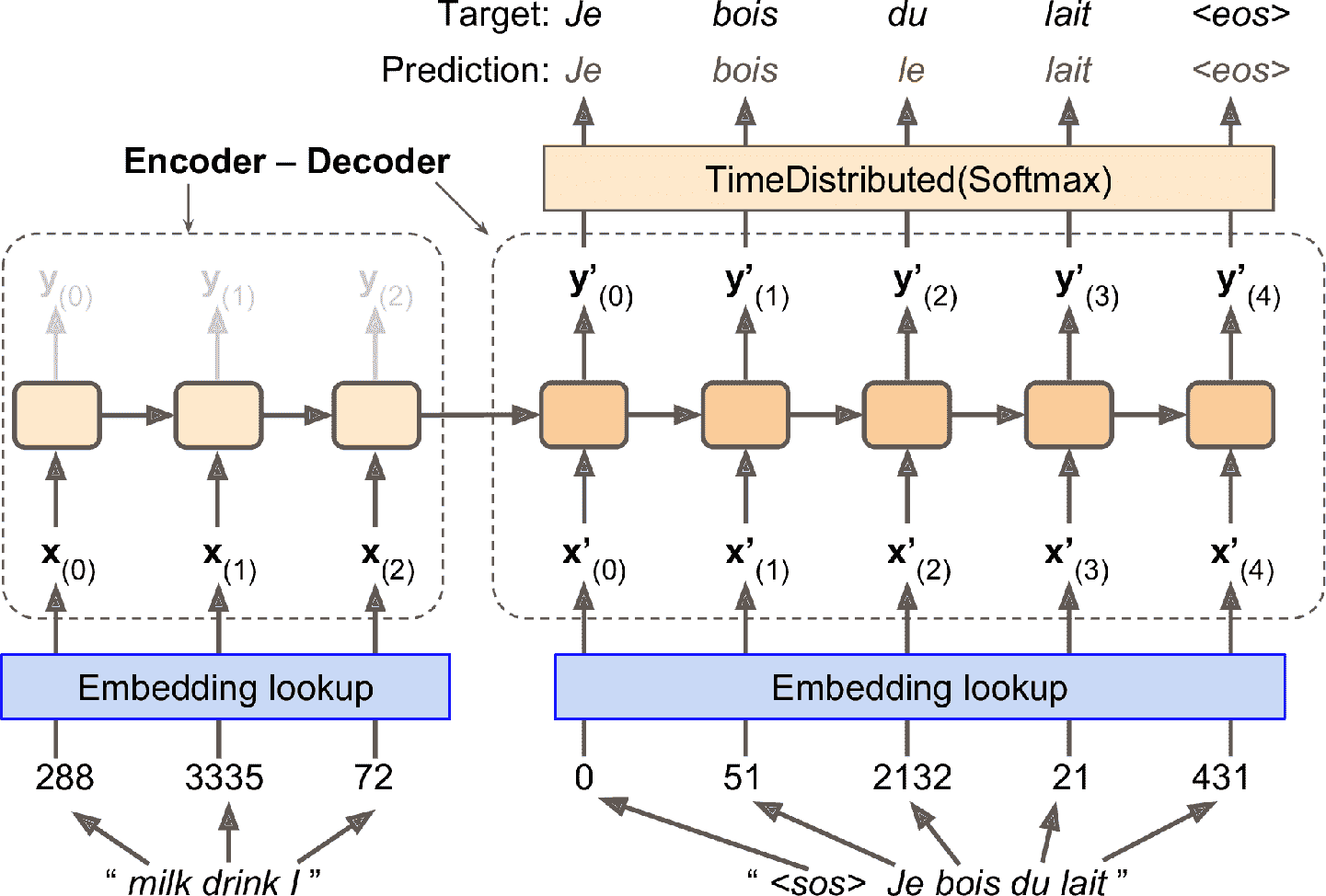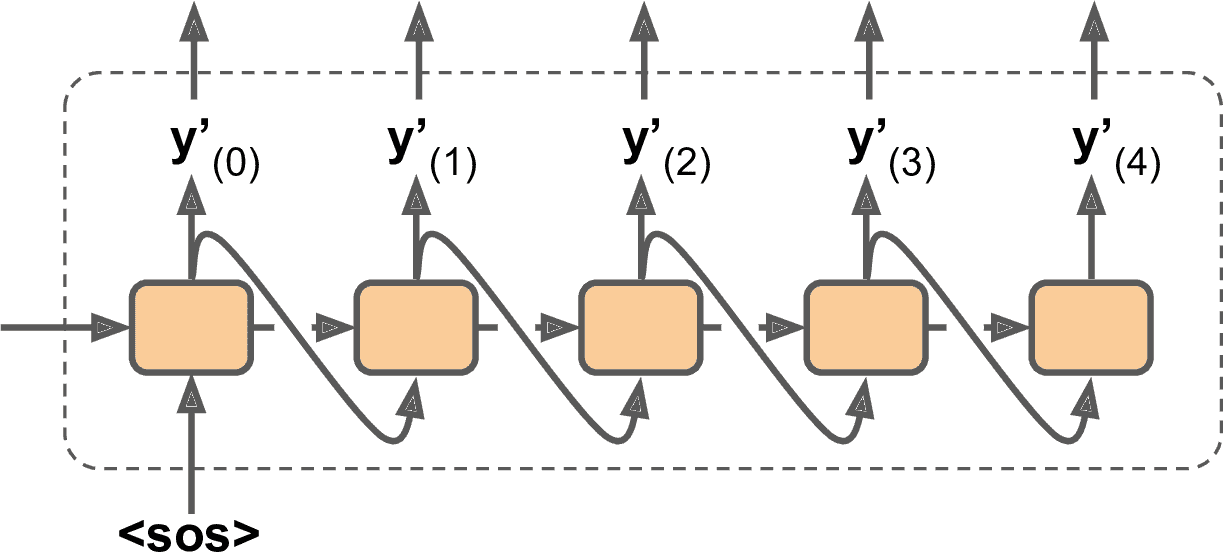• 目前假定所有（编码器和解码器的）输入序列的长度固定。但很显然句子长度是变化的。因为常规张量的形状固定，它们只含有相同长度的句子。可以用遮挡来处理；但如果句子的长度非常不同，就不能像之前情感分析那样截断（因为想要的是完整句子的翻译）。可以将句子放进长度相近的桶里（一个桶放 1 个词到 6 个词的句子，一个桶放 7 个词到 12 个词的句子，等等），给短句子加填充，使同一个桶中的句子长度相同（见tf.data.experimental.bucket_by_sequence_length()函数）。例如，I drink milk变为<pad> <pad> <pad> milk drink I

• 要忽略所有在 EOS 标记后面的输出，这些输出不能影响损失（遮挡起来）。例如，如果模型输出Je bois du lait <eos> oui，忽略最后一个词对损失的影响。

• 如果输出词典比较大（这个例子就是这样），输出每个词的概率会非常慢。如果目标词典有 50000 个发语词，则解码器要输出 50000 维的向量，在这个向量上计算 softmax 非常耗时。一个方法是只查看模型对正确词和非正确词采样的对数概率输出，然后根据这些对数概率计算一个大概的损失。这个采样 softmax 方法是 Sébastien Jean 在 2015 年提出的。在 TensorFlow 中，你可以在训练时使用tf.nn.sampled_softmax_loss()，在推断时使用常规 softmax 函数（推断时不能使用采样 softmax，因为需要知道目标）。

import tensorflow_addons as tfa

encoder_inputs = keras.layers.Input(shape=[None], dtype=np.int32)
decoder_inputs = keras.layers.Input(shape=[None], dtype=np.int32)
sequence_lengths = keras.layers.Input(shape=[], dtype=np.int32)

embeddings = keras.layers.Embedding(vocab_size, embed_size)
encoder_embeddings = embeddings(encoder_inputs)
decoder_embeddings = embeddings(decoder_inputs)

encoder = keras.layers.LSTM(512, return_state=True)
encoder_outputs, state_h, state_c = encoder(encoder_embeddings)
encoder_state = [state_h, state_c]

sampler = tfa.seq2seq.sampler.TrainingSampler()

decoder_cell = keras.layers.LSTMCell(512)
output_layer = keras.layers.Dense(vocab_size)
decoder = tfa.seq2seq.basic_decoder.BasicDecoder(decoder_cell, sampler,
output_layer=output_layer)
final_outputs, final_state, final_sequence_lengths = decoder(
decoder_embeddings, initial_state=encoder_state,
sequence_length=sequence_lengths)
Y_proba = tf.nn.softmax(final_outputs.rnn_output)

model = keras.Model(inputs=[encoder_inputs, decoder_inputs, sequence_lengths],
outputs=[Y_proba])


### 双向 RNN

keras.layers.Bidirectional(keras.layers.GRU(10, return_sequences=True))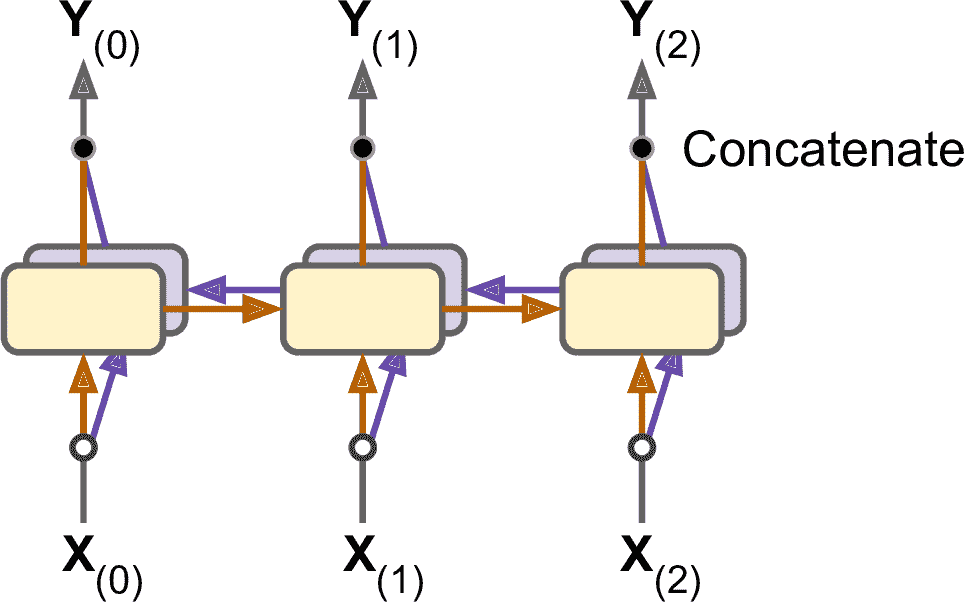### 集束搜索

beam_width = 10
decoder = tfa.seq2seq.beam_search_decoder.BeamSearchDecoder(
cell=decoder_cell, beam_width=beam_width, output_layer=output_layer)
decoder_initial_state = tfa.seq2seq.beam_search_decoder.tile_batch(
encoder_state, multiplier=beam_width)
outputs, _, _ = decoder(
embedding_decoder, start_tokens=start_tokens, end_token=end_token,
initial_state=decoder_initial_state)


## 注意力机制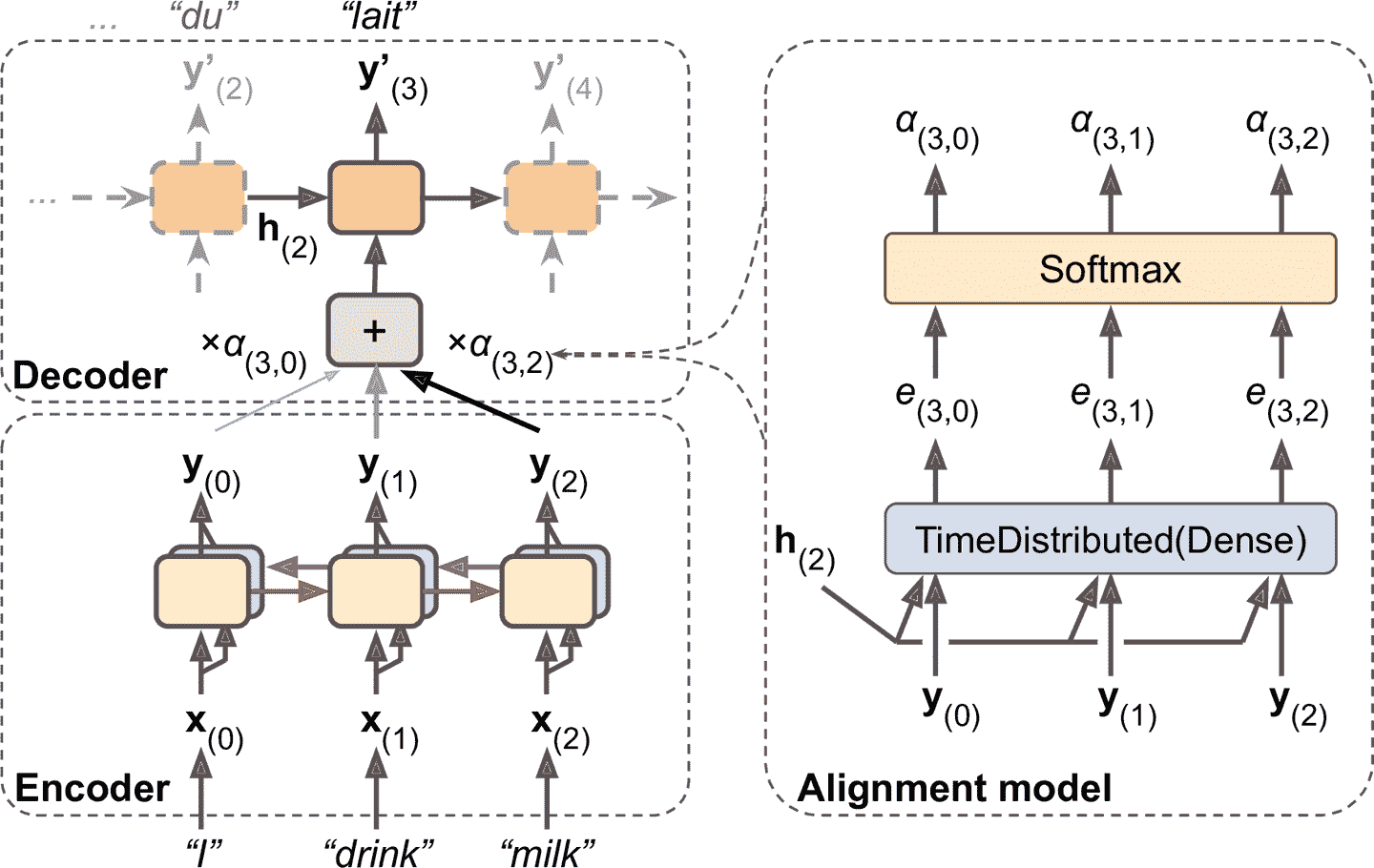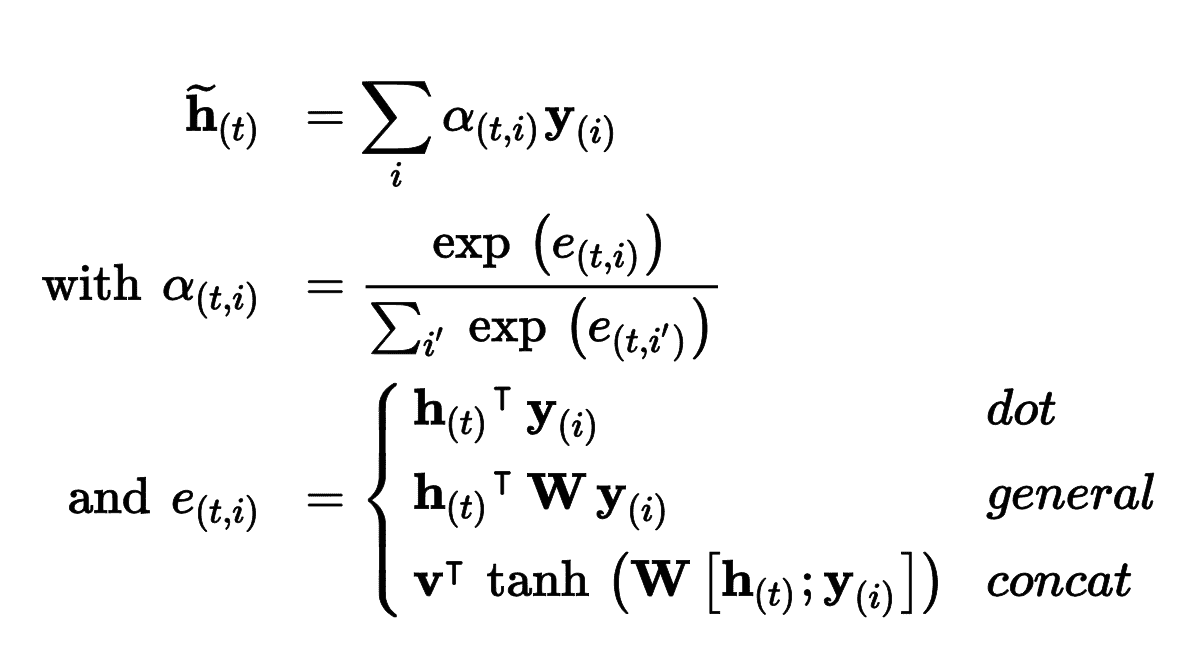attention_mechanism = tfa.seq2seq.attention_wrapper.LuongAttention(
units, encoder_state, memory_sequence_length=encoder_sequence_length)
attention_decoder_cell = tfa.seq2seq.attention_wrapper.AttentionWrapper(
decoder_cell, attention_mechanism, attention_layer_size=n_units)


### 视觉注意力### Attention Is All You Need：Transformer 架构

Transformer 架构见图 16-8。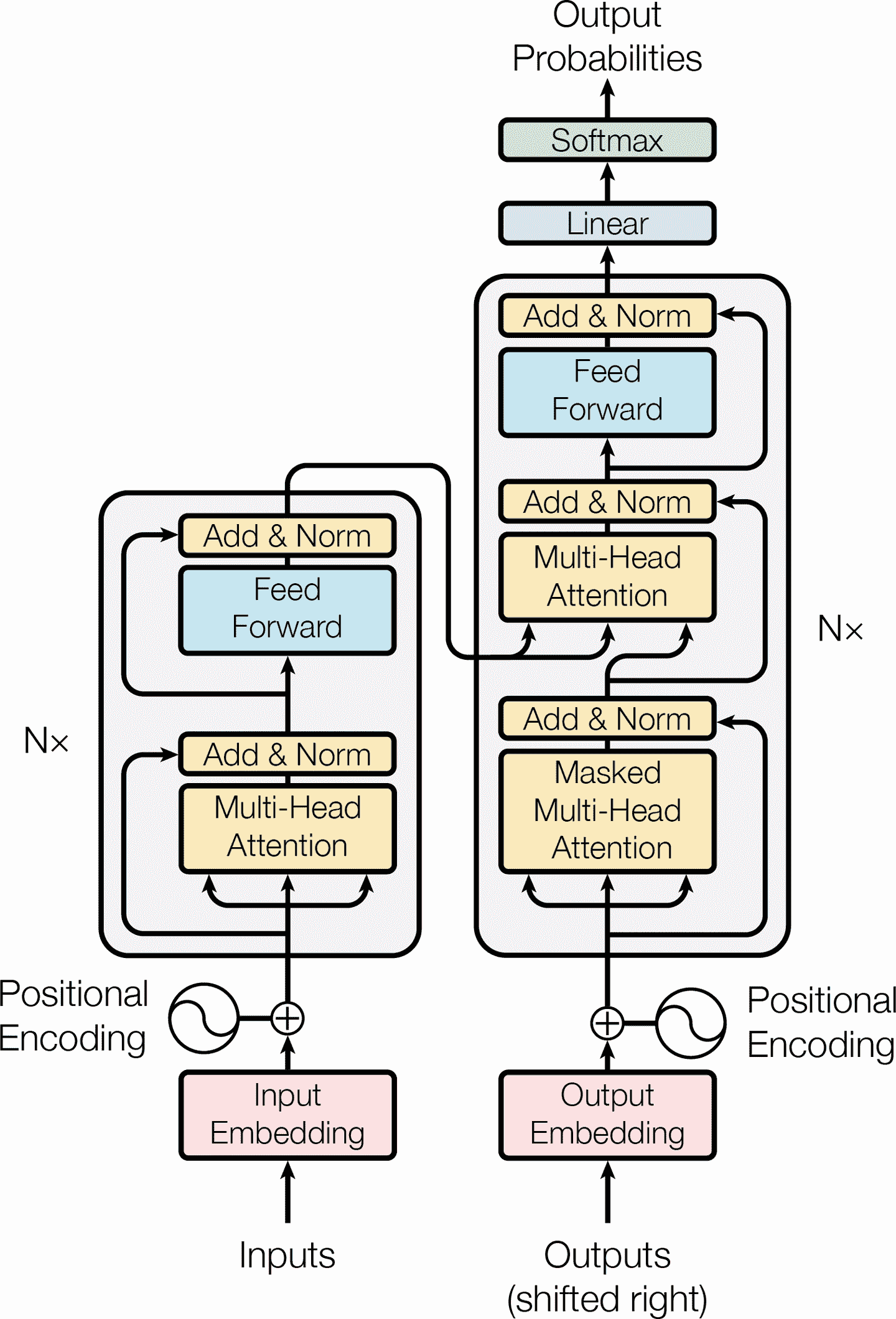• 图的左边和以前一样是编码器，接收的输入是一个批次的句子，表征为序列化的单词 ID（输入的形状是[批次大小, 最大输入句子长度]），每个单词表征为 512 维（所以编码器的输出形状是[批次大小, 最大输入句子长度, 512]）。注意，编码器的头部叠加了N次（论文中，N=6）。

• 架构的右边是解码器。在训练中，它接收目标句子作为输入（也是表征为序列化的单词 ID），向右偏移一个时间步（即，在起点插入一个 SOS 标记）。它还接收编码器的输出（即，来自左边的箭头）。注意，解码器的头部也重叠了N次，编码器的最终输出，传入给解码器重叠层中的每一个部分。和以前一样，在每个时间步，解码器输出每个下一个可能词的概率（输出形状是[批次大小, 最大输出句子长度, 词典长度]）。

• 在推断时，解码器不能接收目标，所以输入的是前面的输出词（起点用 SOS 标记）。因此模型需要重复被调用，每一轮预测一个词（预测出来的词在下一轮输入给解码器，直到输出 EOS 标记）。

• 仔细观察下，可以看到其实你已经熟悉其中大部分组件了：两个嵌入层，5 × N个跳连接，每个后面是一个归一化层，2 × N个“前馈”模块（由两个紧密层组成（第一个使用 ReLU 激活函数，第二个不使用激活函数），输出层是使用 softmax 激活函数的紧密层）。所有这些层都是时间分布的，因此每个词是独立处理的。但是一次只看一个词，该如何翻译句子呢？这时就要用到新组件了：

• 编码器的多头注意力层，编码每个词与句子中其它词的关系，对更相关的词付出更多注意力。例如，输出句子They welcomed the Queen of the United Kingdom中的词Queen的层的输出，会取决于句子中的所有词，但更多注意力会在UnitedKingdom上。这个注意力机制被称为自注意力（句子对自身注意）。后面会讨论它的原理。解码器的遮挡多头注意力层做的事情一样，但每个词只关注它前面的词。最后，解码器的上层多头注意力层，是解码器用于在输入句子上付出注意力的。例如，当解码器要输出Queen的翻译时，解码器会对输入句子中的Queen这个词注意更多。

• 位置嵌入是紧密向量（类似词嵌入），表示词在句子中的位置。第n个位置嵌入，添加到每个句子中的第n个词上。这可以让模型知道每个词的位置，这是因为多头注意力层不考虑词的顺序或位置，它只看关系。因为所有其它层都是时间分布的，它们不知道每个词的（相对或绝对）位置。显然，相对或绝对的词的位置非常重要，因此需要将位置信息以某种方式告诉 Transformer，位置嵌入是行之有效的方法。

### 位置嵌入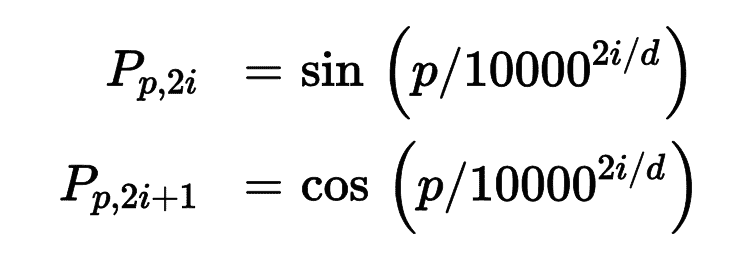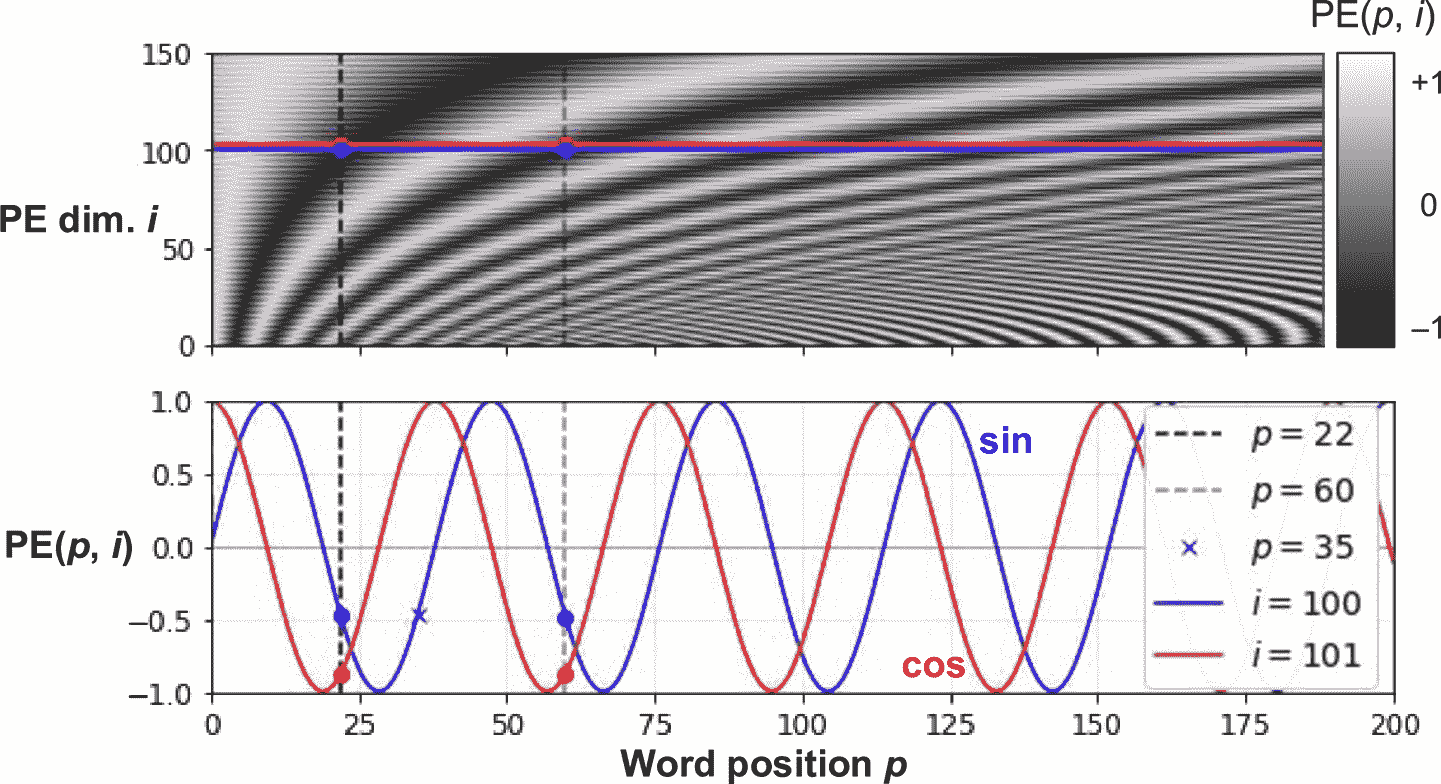TensorFlow 中没有PositionalEmbedding层，但创建很容易。出于效率的考量，在构造器中先计算出位置嵌入（因此需要知道最大句子长度，max_steps，每个词表征的维度，max_dims）。然后调用call()方法裁剪嵌入矩阵，变成输入的大小，然后添加到输入上。因为创建位置嵌入矩阵时，添加了一个大小为 1 的维度，广播机制可以确保位置矩阵添加到输入中的每个句子上：

class PositionalEncoding(keras.layers.Layer):
def __init__(self, max_steps, max_dims, dtype=tf.float32, **kwargs):
super().__init__(dtype=dtype, **kwargs)
if max_dims % 2 == 1: max_dims += 1 # max_dims must be even
p, i = np.meshgrid(np.arange(max_steps), np.arange(max_dims // 2))
pos_emb = np.empty((1, max_steps, max_dims))
pos_emb[0, :, ::2] = np.sin(p / 10000**(2 * i / max_dims)).T
pos_emb[0, :, 1::2] = np.cos(p / 10000**(2 * i / max_dims)).T
self.positional_embedding = tf.constant(pos_emb.astype(self.dtype))
def call(self, inputs):
shape = tf.shape(inputs)
return inputs + self.positional_embedding[:, :shape[-2], :shape[-1]]


embed_size = 512; max_steps = 500; vocab_size = 10000
encoder_inputs = keras.layers.Input(shape=[None], dtype=np.int32)
decoder_inputs = keras.layers.Input(shape=[None], dtype=np.int32)
embeddings = keras.layers.Embedding(vocab_size, embed_size)
encoder_embeddings = embeddings(encoder_inputs)
decoder_embeddings = embeddings(decoder_inputs)
positional_encoding = PositionalEncoding(max_steps, max_dims=embed_size)
encoder_in = positional_encoding(encoder_embeddings)
decoder_in = positional_encoding(decoder_embeddings)


### 多头注意力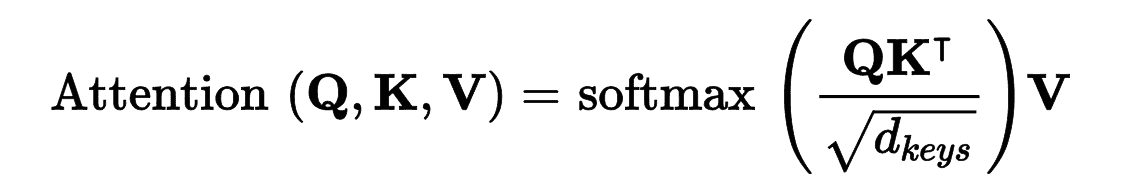• Q矩阵每行是一个查询词。它的形状是[n_queries, d_keys]n_queries是查询数，d_keys是每次查询和每个键的维度数。

• K矩阵每行是一个键。它的形状是[n_keys, d_keys]n_keys是键和值的数量。

• V矩阵每行是一个值。它的形状是[n_keys, d_values]d_values是每个值的数。

• Q K^T的形状是[n_queries, n_keys]：它包含这每个查询/键对的相似分数。softmax 函数的输出有相同的形状，且所有行的和是 1。最终的输出形状是[n_queries, d_values]，每行代表一个查询结果（值的加权和）。

• 缩放因子缩小了相似度分数，防止 softmax 函数饱和（饱和会导致梯度变小）。

• 在计算 softmax 之前，通过添加一些非常大的负值，到对应的相似度分上，可以遮挡一些键值对。这在遮挡多头机制层中很有用。

keras.layers.Attention层实现了缩放点积注意力，它的输入是QKV，除此之外，还有一个批次维度（第一个维度）。

Z = encoder_in
for N in range(6):
Z = keras.layers.Attention(use_scale=True)([Z, Z])

encoder_outputs = Z
Z = decoder_in
for N in range(6):
Z = keras.layers.Attention(use_scale=True, causal=True)([Z, Z])
Z = keras.layers.Attention(use_scale=True)([Z, encoder_outputs])

outputs = keras.layers.TimeDistributed(
keras.layers.Dense(vocab_size, activation="softmax"))(Z)


use_scale=True参数可以让层学会如何缩小相似度分数。这是和 Transformer 的一个区别，后者总是用相同的因子（）缩小相似度分数。causal=True参数，可以让注意力层的每个输出标记只注意前面的输出标记。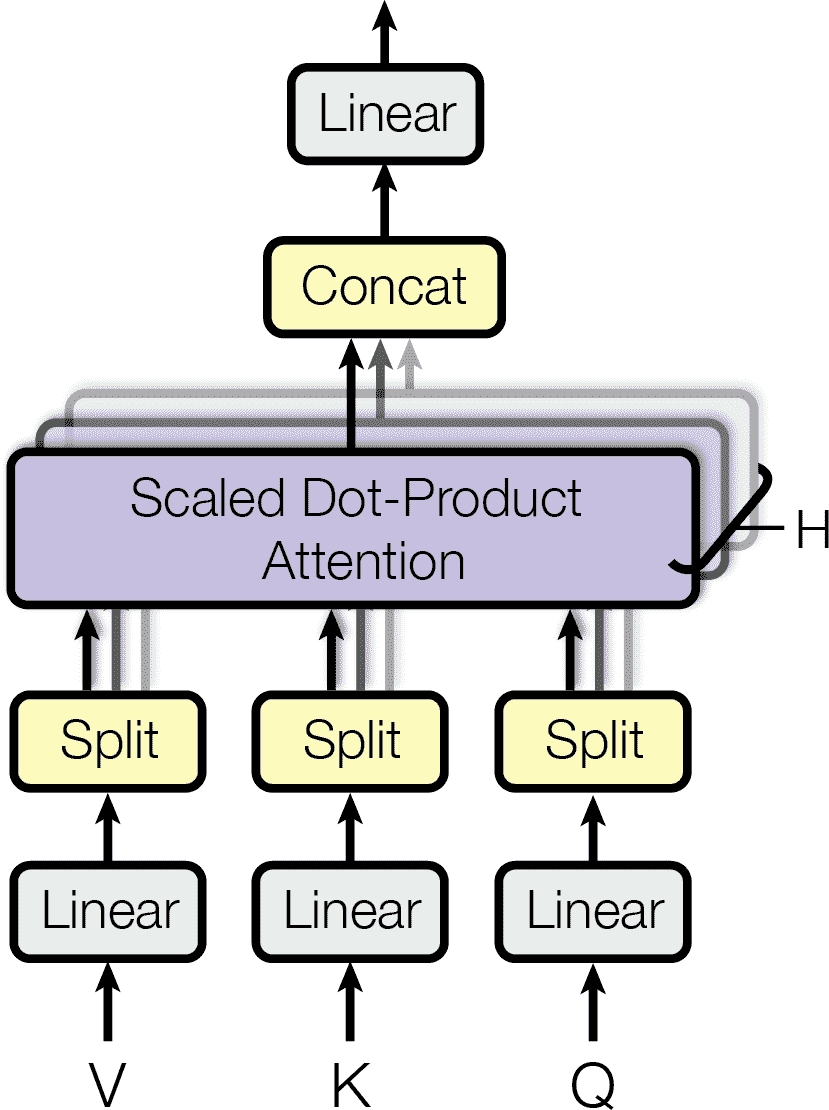## 语言模型的最新进展

2018 年被称为“NLP 的 ImageNet 时刻”：成果惊人，产生了越来越大的基于 LSTM 和 Transformer、且在大数据集上训练过的架构。建议你看看下面的论文，都是 2018 年发表的：

• Matthew Peters 的 ELMo 论文，介绍了语言模型的嵌入（Embeddings from Language Models (ELMo)）：学习深度双向语言模型的内部状态，得到的上下文词嵌入。例如，词QueenQueen of the United Kingdomqueen bee中的嵌入不同。

• Jeremy Howard 和 Sebastian Ruder 的 ULMFiT 论文，介绍了无监督预训练对 NLP 的有效性：作者用海量语料，使用自监督学习（即，从数据自动生成标签）训练了一个 LSTM 语言模型，然后在各种任务上微调模型。他们的模型在六个文本分类任务上取得了优异的结果（将误差率降低了 18-24%）。另外，他们证明，通过在 100 个标签样本上微调预训练模型，可以达到在 10000 个样本上训练的效果。

• Alec Radford 和其他 OpenAI 人员的 GPT 论文，也展示了无监督训练的有效性，但他们使用的是类似 Transformer 的架构。作者预训练了一个庞大但简单的架构，由 12 个 Transformer 模块组成（只使用了遮挡多头注意力机制），也是用自监督训练的。然后在多个语言任务上微调，只对每个任务做了小调整。任务种类很杂：包括文本分类、衔接（句子 A 是否跟着句子 B），相似度（例如，Nice weather todayIt is sunny很像），还有问答（通过阅读几段文字，让模型来回答多选题）。几个月之后，在 2019 年的二月，Alec Radford、Jeffrey Wu 和其它 OpenAI 的人员发表了 GPT-2 论文，介绍了一个相似的架构，但是更大（超过 15 亿参数），他们展示了这个架构可以在多个任务上取得优异的表现，且不需要微调。这被称为零次学习（zero-shot learning (ZSL)）。这个页面上是一个 GPT-2 模型的带有预训练权重的小型版本，“只有”1.17 亿个参数。

• Jacob Devlin 和其它 Google 人员的 BERT 论文，也证明了在海量语料上做自监督预训练的有效性，使用的是类似 GPT 的架构，但用的是无遮挡多头注意力层（类似 Transformer 的编码器）。这意味着模型实际是双向的这就是 BERT（Bidirectional Encoder Representations from Transformers）中的 B 的含义。最重要的，作者提出了两个预训练任务，用以测试模型能力：

遮挡语言模型（MLM） 句子中的词有 15 的概率被遮挡。训练模型来预测被遮挡的词。例如，如果原句是She had fun at the birthday party，模型的输入是She <mask> fun at the <mask> party，让模型来预测hadbirthday（忽略其它输出）。更加准确些，每个选出的单词有 80% 的概率被遮挡，10% 的概率被替换为随机词（降低预训练和微调的差异，因为模型在微调时看不到<mask>标记），10% 的概率不变（使模型偏向正确答案）。

预测下一句（NSP） 训练模型预测两句话是否是连续的。例如，模型可以预测The dog sleepsIt snores loudly是连续的，但是The dog sleepsThe Earth orbits the Sun是不连续的。这是一个有挑战的任务，可以在微调任务，比如问答和衔接上，极大提高模型的性能。

## 练习

1. 有状态 RNN 和无状态 RNN 相比，优点和缺点是什么？

2. 为什么使用编码器-解码器 RNN，而不是普通的序列到序列 RNN，来做自动翻译？

3. 如何处理长度可变的输入序列？长度可变的输出序列怎么处理？

4. 什么是集束搜索，为什么要用集束搜索？可以用什么工具实现集束搜索？

5. 什么是注意力机制？用处是什么？

6. Transformer 架构中最重要的层是什么？它的目的是什么？

7. 什么时候需要使用采样 softmax？

8. Hochreiter 和 Schmidhuber 在关于 LSTM 的论文中使用了嵌入 Reber 语法。这是一种人工的语法，用来生成字符串，比如BPBTSXXVPSEPE。查看 Jenny Orr 对它的介绍。选择一个嵌入 Reber 语法（比如 Jenny Orr 的论文中展示的），然后训练一个 RNN 来判断字符串是否符合语法。你需要先写一个函数来生成训练批次，其中 50% 符合语法，50% 不符合语法。

9. 训练一个编码器-解码器模型，它可以将日期字符串从一个格式变为另一个格式（例如，从April 22, 2019变为2019-04-22）。

10. 阅读 TensorFlow 的《Neural Machine Translation with Attention tutorial》

11. 使用一个最近的语言模型（比如，BERT），来生成一段更具信服力的莎士比亚文字。

{% endraw %}